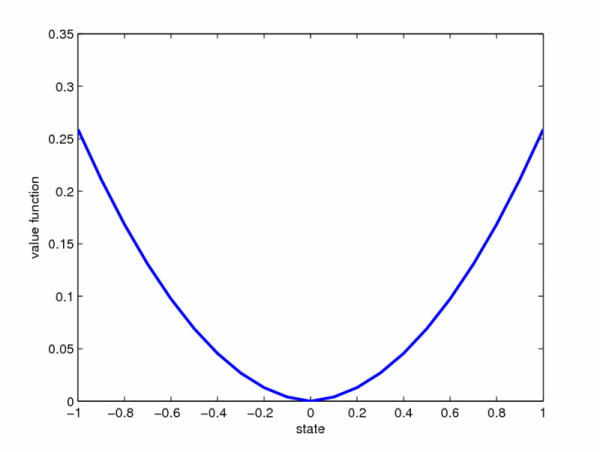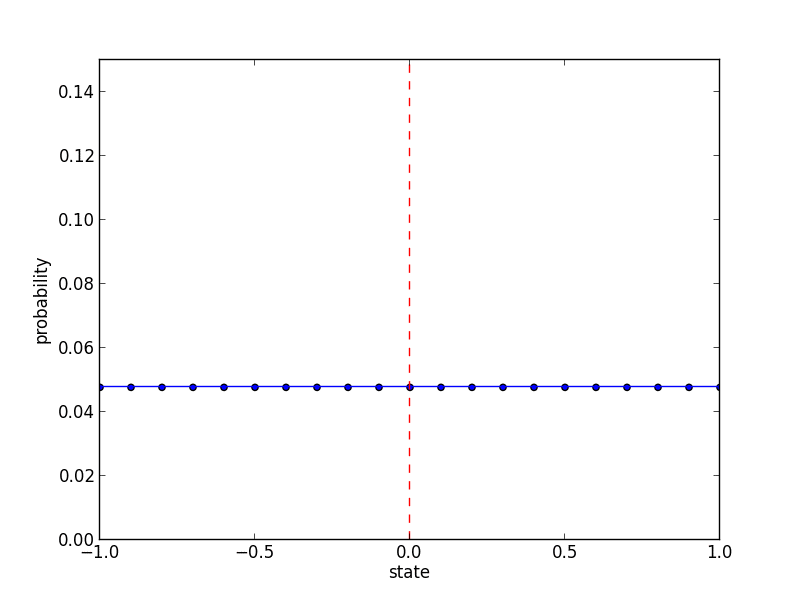# 1D Integrator

Here is a simple deterministic problem, without discrete modes for for illustrating the process law. We consider a classical 1D integrator.$Latex formula$

The following figure shows the value function at initial time. As expected, the value function is 0 when x=0, and increases symmetrically when getting farther form it.We set the state probabilities at initial time according to a discrete uniform distribution$Latex formula$

The following animated figure shows the evolution of the state probabilities over the grid for each time step. We observe a concentration of the distribution towards x=0.